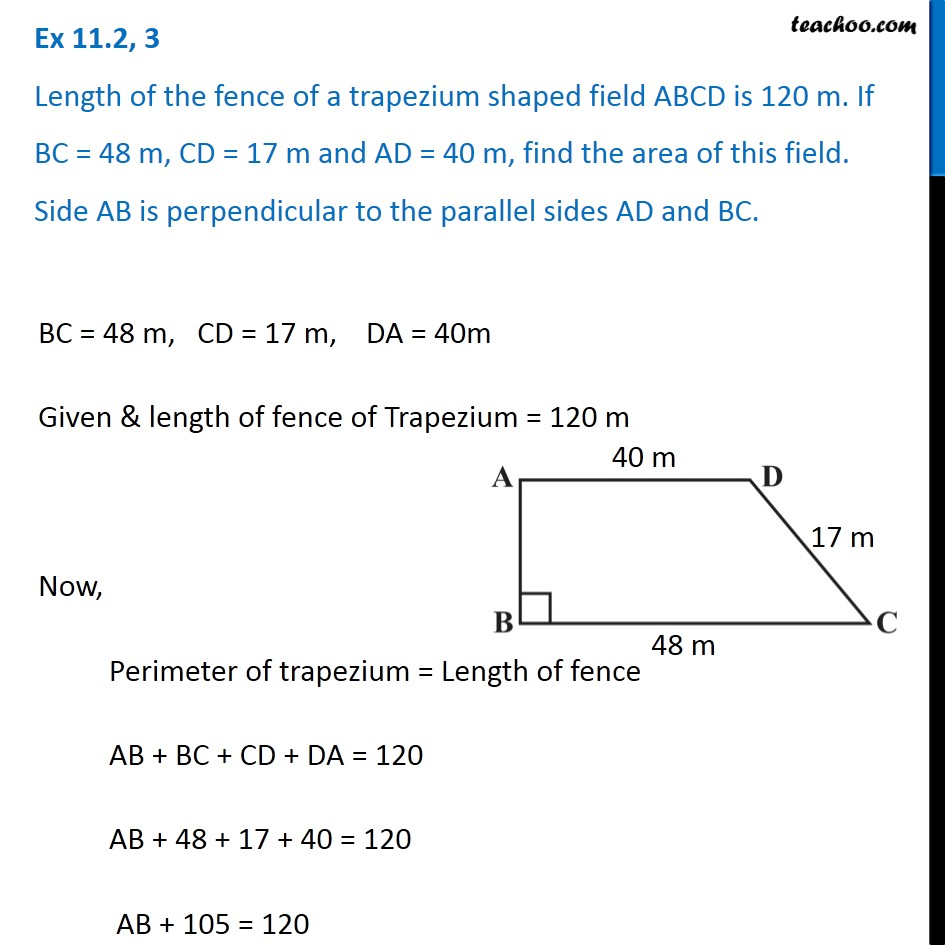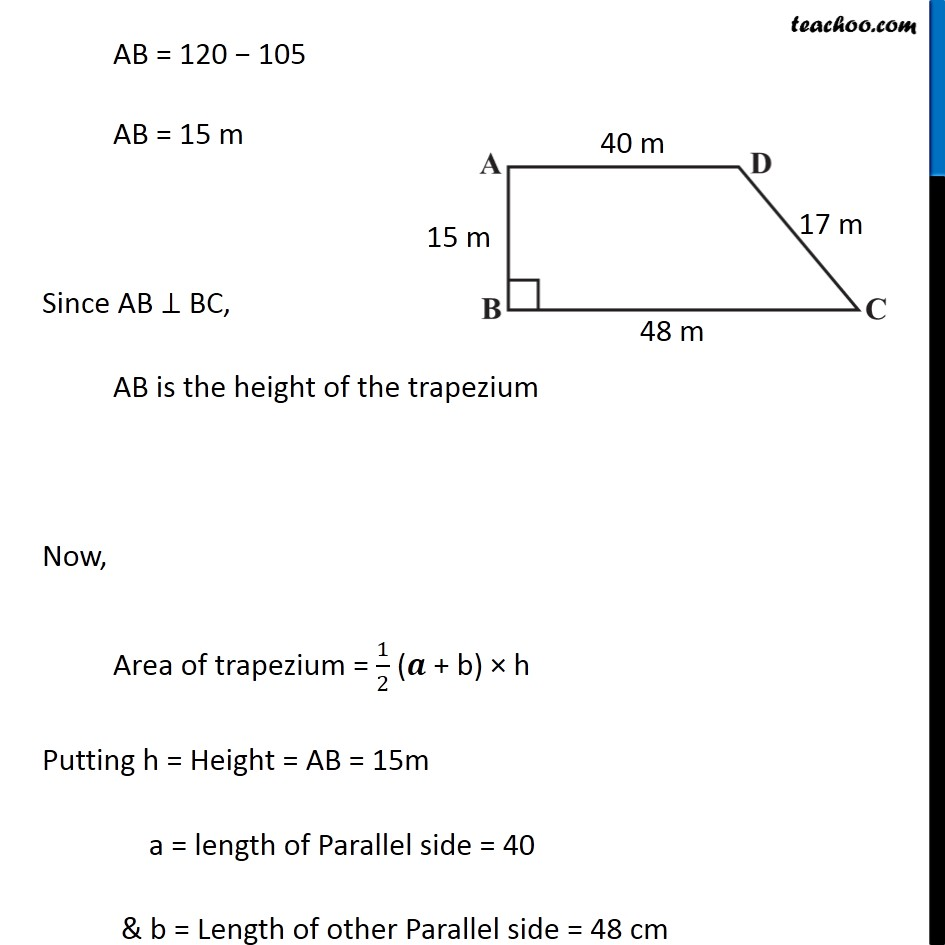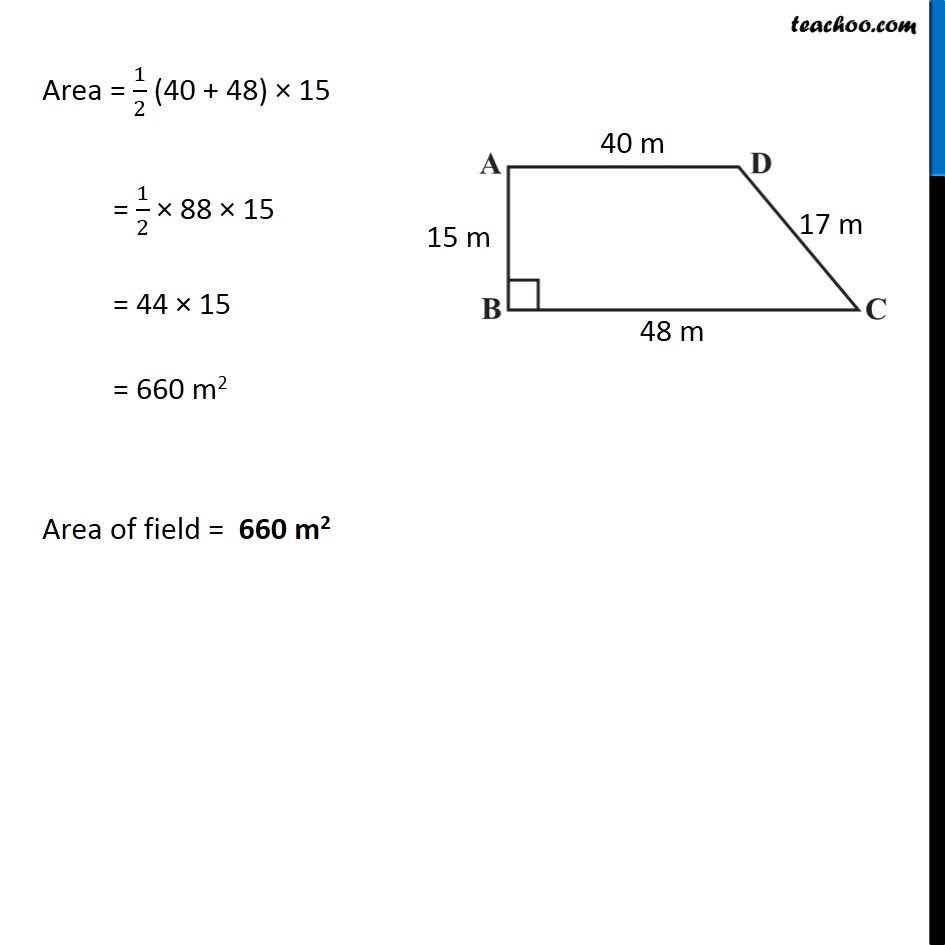Subscribe to our Youtube Channel - https://you.tube/teachoo

1. Chapter 11 Class 8 Mensuration
2. Concept wise
3. Area of Trapezium

Transcript

Ex 11.2, 3 Length of the fence of a trapezium shaped field ABCD is 120 m. If BC = 48 m, CD = 17 m and AD = 40 m, find the area of this field. Side AB is perpendicular to the parallel sides AD and BC. BC = 48 m, CD = 17 m, DA = 40m Given & length of fence of Trapezium = 120 m Now, Perimeter of trapezium = Length of fence AB + BC + CD + DA = 120 AB + 48 + 17 + 40 = 120 AB + 105 = 120 AB = 120 − 105 AB = 15 m Since AB ⊥ BC, AB is the height of the trapezium Now, Area of trapezium = 1/2 (𝒂 + b) × h Putting h = Height = AB = 15m a = length of Parallel side = 40 & b = Length of other Parallel side = 48 cm Area = 1/2 (40 + 48) × 15 = 1/2 × 88 × 15 = 44 × 15 = 660 m2 Area of field = 660 m2

Area of Trapezium